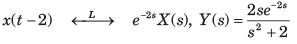Courses

# Test: Laplace Part - 3

## 10 Questions MCQ Test Signal and System | Test: Laplace Part - 3

Description
This mock test of Test: Laplace Part - 3 for Electrical Engineering (EE) helps you for every Electrical Engineering (EE) entrance exam. This contains 10 Multiple Choice Questions for Electrical Engineering (EE) Test: Laplace Part - 3 (mcq) to study with solutions a complete question bank. The solved questions answers in this Test: Laplace Part - 3 quiz give you a good mix of easy questions and tough questions. Electrical Engineering (EE) students definitely take this Test: Laplace Part - 3 exercise for a better result in the exam. You can find other Test: Laplace Part - 3 extra questions, long questions & short questions for Electrical Engineering (EE) on EduRev as well by searching above.
QUESTION: 1

### Determine the time signal x(t) corresponding to given X (s) and choose correct option. Q.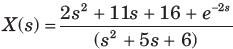Solution: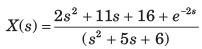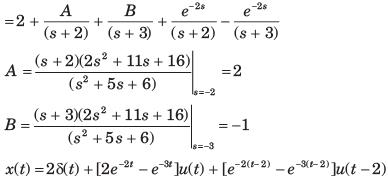QUESTION: 2

### Determine the time signal x(t) corresponding to given X (s) and choose correct option. Q.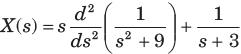Solution: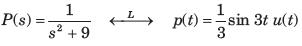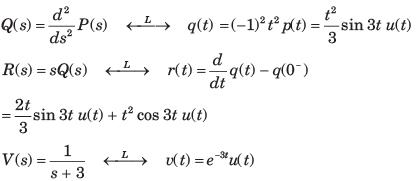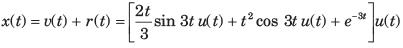QUESTION: 3

### Determine the time signal x(t) corresponding to given X (s) and choose correct option. Q.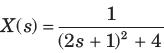Solution: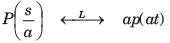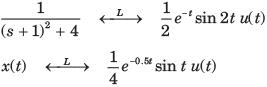QUESTION: 4

Determine the time signal x(t) corresponding to given X (s) and choose correct option.
Q.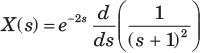Solution: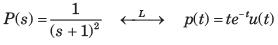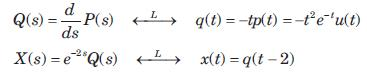x(t) =  -( t - 2) 2e-(t-2) u( t - 2)

QUESTION: 5

Given the transform pair below. Determine the time signal y(t) and choose correct option.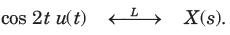Q. Y (s) = ( s + 1) X ( s)

Solution: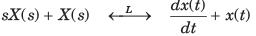⇒ y(t) = ( -2 sin2 t + cos 2 t ) u( t)

QUESTION: 6

Given the transform pair below. Determine the time signal y(t) and choose correct option.Q. Y (s) = X ( 3s)

Solution: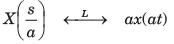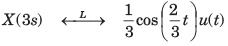QUESTION: 7

Given the transform pair below. Determine the time signal y(t) and choose correct option.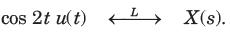Q. Y (s) = X ( s + 2)

Solution: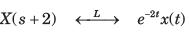QUESTION: 8

Given the transform pair below. Determine the time signal y(t) and choose correct option.Q.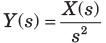Solution: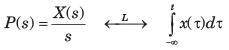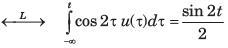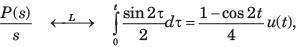QUESTION: 9

Given the transform pair below. Determine the time signal y(t) and choose correct option.Q.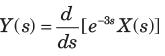Solution: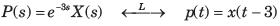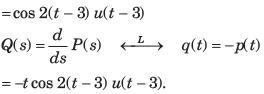QUESTION: 10

Given the transform pair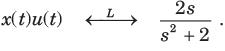Determine the Laplace transform Y (s) of the given time signal in question and choose correct option.
Q. y(t) = x(t - 2)

Solution: